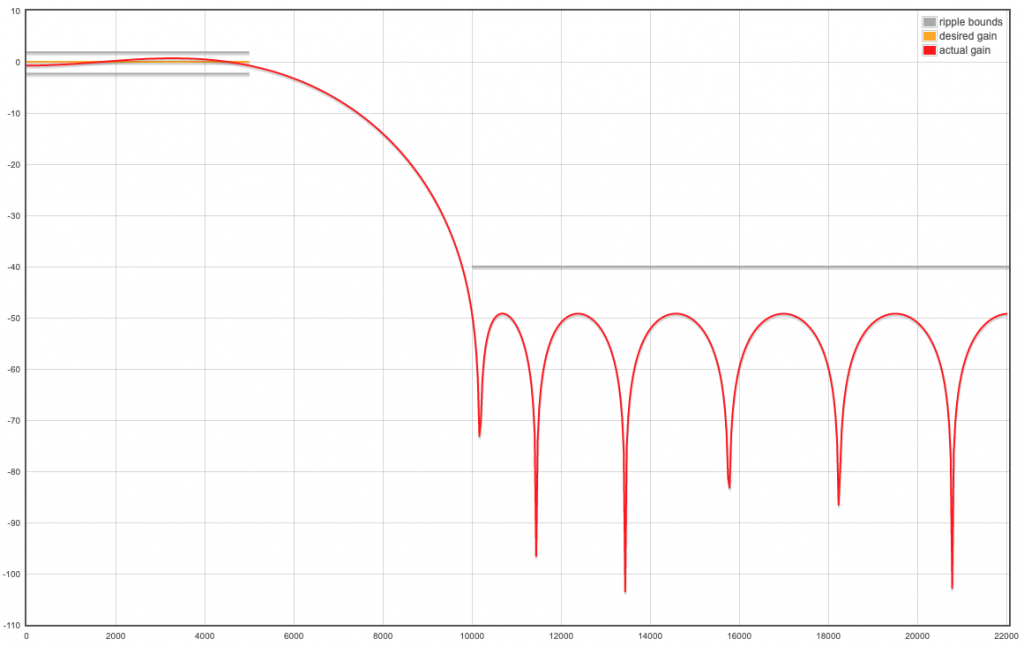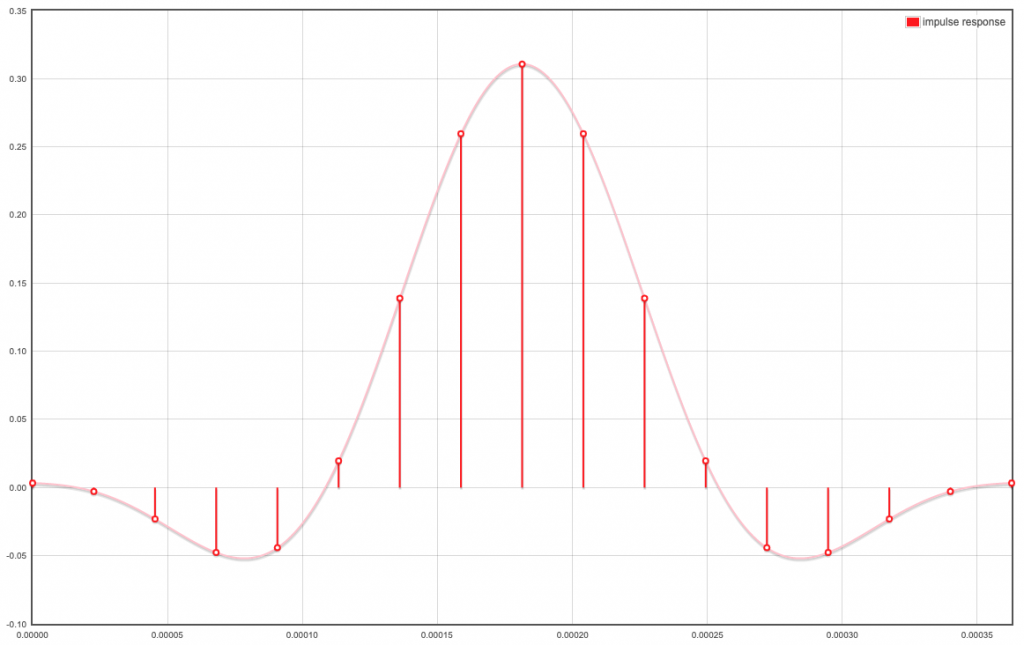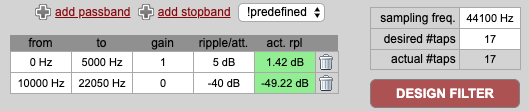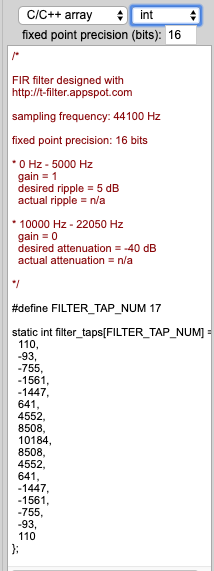# APU Configuration

• Hi,

I'd like to use the APU to beamform with frequency content higher than voice. The datasheet suggests that the sample rate can be up to 192kHz. Is that for each individual I2S channel?

In the standalone SDK I found the APU demo and in init.c I found...

``````i2s_set_sample_rate(I2S_DEVICE_0, 44100);
``````

...so it seems I can increase the sample rate. That's one piece of the puzzle.

Next in the same file comes code to set FIR filter coefficients. For example:

``````uint16_t fir_prev_t[] = {
0x020b, 0x0401, 0xff60, 0xfae2, 0xf860, 0x0022,
0x10e6, 0x22f1, 0x2a98, 0x22f1, 0x10e6, 0x0022,
0xf860, 0xfae2, 0xff60, 0x0401, 0x020b,
};
``````

There are 4 such definitions with no indication of how the coefficients have been determined. To get the beamformer running at higher frequencies I will absolutely need to know how to determine the coefficients because I assume the existing coefficients determine a cutoff frequency close to 22kHz. I need to see a schematic or block diagram for the filters used in the APU and the formulas used to define the FIR filters to move forward.

I'd also like to better understand how the beamformer works. From a review of the library and demo code I think I understand that the BF hardware returns 16 vectors with each vector corresponding to a different direction ahead of the microphone array with the content being N samples of frequency/amplitude pairs, which are the results of the FFT performed in each of the 16 directions. Does that sound right?

What I can't easily tell from the code is how the diretion vectors are determined? Each vector would orginate from the center of the array but how do I determine the elevation and azimuth of each vector so I can identify what they are "looking" at?

Would this be representative?... 3d PDF >>> here <<< You will need to download the PDF into a 3D capable viewer as it may not render in a browser.

I need to know how the vector geometry is determined and how to configure the filters.

A guide or at least additional detail on the hardware would be helpful. I have already translated the "Kendryte Standalone SDK Programming Guide [Simplified Chinese]" document to English and I see that there is a section on the APU but it doesn't address my questions. Can anyone assist with this?

Thanks!

• @spblinux Update: Code shown in blog has been refactored and compiles now in Arduino IDE and in standalone SDK.

• @spblinux Some progress is described here. 7 channel input at 88kHz sample rate is working producing output with 44kHz sample rate.
Had to cope with i2s sample rates. Voice output requires that input rate is exactly an integer multiple of output rate. Failed to set it with function i2s_set_sample_rate because of rounding errors. Had to use function sysctl_clock_set_threshold directly to define i2s clock frequency. Furthermore it seems to be required to use downsampling by at least factor 2 for apu_voc: apu_set_down_size(0, 1).
Had to learn that: i2s_clock_frequency == i2s_sample_rate * sysclk_cycles_bit_width * 2 * number_of_stereo_channels_used

• I'm exploring the apu demo further and to see if I can understand better how it determines the directon to the source of sound. I know that it calculates the square of all elements of the array called APU_DIR_BUFFER, which is defined as...

``````extern int16_t APU_DIR_BUFFER[APU_DIR_CHANNEL_MAX]
[APU_DIR_CHANNEL_SIZE]
__attribute__((aligned(128)));
``````

APU_DIR_CHANNEL_MAX = 16
APU_DIR_CHANNEL_SIZE = 512

... , adds them together and divides by APU_DIR_CHANNEL_SIZE to get a squared average. Earlier I guessed that the APU H/W performed an FFT on the beam-focussed signal in each of the 16 directions so the RMS calculation was operating on frequencies but this doesn't seem to be the case.

I printf'd all elements of the buffer to the console expecting it to show higher values <5kHz (because of the 5kHz LP FIR filter) but it doesn't look like that. Instead I see about 50% of the values being negative so this can't be frequency data. If it isn't frequency data then it might be something distance related.

@manageryzy included a block in their data flow diagram that was labelled "DAS BF", which stands for "Delay and Sum Beamformer. I should have caught this sooner because I now recall that a delay and sum beamformer works by time shifting the sound samples from each mic to compare with the others. For a given mic-to-mic comparison the undelayed signal from one mic will add constructively with one of the delayed sequences from the 2nd mic and the summation of the result will be greater than the same summation for two other mics. For a given microphone geometry the amount of delay necessary is readily determined. Theory for uniform circular arrays >>> here <<<

If you go to section 4.3 Delay&Sum Beamformer on PDF page #47 you will find a fairly simply description of the implementatin and nowhere does it indicate a squaring operation is necessary. I found this confusing so I experiemented with the code and tried things like removing the squaring operation but the operation completely broke down. This suggestes to me that the beamformer is a different type, perhaps the "Robust Least Squares (LS) Frequency Invariant Beamformer", which is dscribed in the mentioned text. The problem is that the LS method seeks to minimize the sum of squares operation and the apu demo is working with the maximum value. I spent a day on this and I can't explain this apparent contradiction. I accept that it works but I am frustrated by the lack of technical information available to the developing community.

I'll share another useful tip. From my last video you would have noticed that most of the time the histrogram/chart is smoothly rising and falling on either side of the best guess direction to the source but sometimes the beamformer breaks down and loses its ability to track the source. You can programmatically identify when the beamformer is broken by using the fact that the top 3-5 or whatever directions vectors which are centered on the source will have sequential numbers... For example if channel #11 is strongest then you would see the chart rise from 9 through 10 to 11 and fall to 13 through 12. When more channels follow this pattern you would have greater confidence in the direction to source. Likewise if their were fewer with this pattern, with one in the extreme, then you could interpret that as an unstable beamformer and take steps to remedy it (wait or reset device for example).

When things are working well you could probably generate a better angle to source estimate with a weighted average as SUM(angle[i]*value[i])/SUM(value[i]). I'll experiment with this when I have time. There is also a way to determine which of the dominate directions are in sequence by using the formula for the sum of a sequence (1, 2, 3, ... n) = n(n+1)/2. The mathy types out there might explore that. If I'm able to implement that I'll share a video.

Still moving forward.

• @MyAmigo I am interested in voice processing, so up to 4kHz should be ok. However sound running through APU is accompagnied by quite a few APU induced noises. Looking back to analog times: there was some white noise of high frequency and some 50Hz/60Hz noise.

With APU you have samples, aka pieces of sound waves. And, at least for me, it was not obvious to find settings which put the pieces continuously together. Downsampling API_VOC by factor 2, did help a lot to remove glitches. I guess I was coping with somehow produced delays. (Have to find time to put code examples on my blog.). - Next week I have no access to my K210 board (maix go). Then I will continue.

• @spblinux I doubt you'll get anything useful with frequency content greater than 4kHz due to spatial aliasing effects. I suggest you investigate and add a bit about that to your blog and summarize here.

4cm spacing with the 6+1 mic array is limiting factor. Generate coefficients for a 4 or 5kHz LP filter and assign to both the PREV and POST FIR filter stages. The existing POST FIR filter is notched at 5kHz and rolls off on either side.

Good luck!

• @MyAmigo some of my findings are here (to be continued); trying to get usable APU_VOC output from 6+1 mic array. - Direction detection with 440hz sine wave works fine, led on mic array showing direction of sound ...

• Here’s an updated video with the original 5kHz low pass filter in the device and a 2kHz tone. This is a much better demo than the last one.

https://photos.app.goo.gl/VvP6rKaahKcAmLwU6

• Ok so I'll share a little of what I've learned. The alternate filters seem to work. I tested a 10kHz signal on the default 5kHz LP filter and of course that was blocked. A filter with a higher cutoff frequency allowed higher frequency content through as expected. I even tested a 12kHz - 20kHz signal and the program ignored my voice while it was running. When I switched on an 18kHz source (tone generator on my phone) it got busy with bigger numbers. I found the numbers difficult to interpret since they were flying by the screen at high speed so I modified the apu demo files to generate a simple bar graph. I recorded a video >>> here <<<.

Setup...

• Using Sipeeds's 6+1 mic array
• Configured with 12-20kHz BP filter coefficients on PREV & POST FIR filters
• Fstop1 = 7kHz, Fpass1 = 12kHz, Fpass2 = 20kHz & Fstop2 = 25kHz
• I2S_SF define in apu.h changed to 54000 (54kHz) so that satisfied the 25kH Fstop
• Demo init.c uses I2S_SF instead of hardcoded value
• I disabled the VOC logic (set APU_VOC_ENABLE = 0)
• Adjusted parameters in function: apu_set_delay(4, 6, 0)... 4cm mic spacing with 6+1 array PCB; 6 mics in circle; ignore center mic

Observations:

• The relationship of the energy detected in one direction bar on the graph relative to the next doesn’t match what I thought it would.
• This is likely because the mic spacing is now too great for the test signal so the beamformer is not working.
• The Wavelength at 18kHz is 342/18000 = 0.019m = 1.9cm and we know that the spacing has to be less than ½ this value to avoid spatial aliasing (look it up) so ~1cm.
• The current spacing is 4cm so we should expect it to not work.

• The default demo came with a 5kHz LP filter.
• The wavelength of a 5kHz signal is 342/5000 = .068m = 6.8cm so the spacing should be less than 3.4cm.
• The spacing for the 6+1 array is actually 4cm so testing the default demo with a marginally 5kHz signal should produce inconsistent results.
• This probably explains why the demo was setup with a 5khz LP FIR filter... it might not work otherwise and that would make for a terrible demo

Getting there!

• @spblinux ... nice to see someone else pondering this topicI see that you have roughly the same magnitude for your coefficients as I do. I also note that the coeffecients from the BF demo code indicate a passband attentuation of ~6dB. I haven't seen a filter design tool that would also allow you to specify attenuation but a filter can be attenuated by applying a common scale factor to all coefficients. The DC gain of a filter is the sum of the coefficients, which totals 0.51532 for the BF demo. Dividing each coefficient by this number shifts the magnitude response up so there is 0 attenuation in the passband. Now we can see that a filter generated using filterDesigner needs to have a stopband attenuation of ~30dB...... so we can try different settings with our original attempt at replicating the BF coefficients and when we get this response we only need to scale the coefficients to determine values that best match the original BD demo filter.

I found that changing the Wstop parameter to 0.2928 produced a mag plot that was almost identical to the above...By then scaling the coefficients so the gain of this filter matches the original BF demo coefficients we get virtually the same mag plot as the BF demo.The RMS error between the original BF demo coefficients and these new ones after setting Wstop=0.2928 and scaling is 0.3% so that's close enough for me.

I think I can start experimenting with filter settings now.

• @MyAmigo Great! That is one more step to deal with the apu.
For all those without a copy of matlab. At http://t-filter.engineerjs.com/ very similar filter coefficients can be created:TFilter uses int16_t numbers, sdk example apu/init.c uses uint16_t.

• @manageryzy thank you. I thought the common coefficients were there to apply gain. I can confirm whether that is actually used by changing the values and seeing if it affects the performance of the hardware. Speaking of gain... there is a hardware function called audio_bf_set_audio_gain which I assume is adjusted to correct overall gain for the system so it is applied to all frequencies. Does that sound right?

I figured out how to generate a filter in Matlab using just the coefficients so I loaded the 17 coefficients from the BF demo into Matlab (after converting to fixed-point real numbers) and obtained the following impulse reponse (to confirm import)...and the resulting magnitude response...... assuming FS=48kHz. This is helpful as it suggests a Fpass of about 5kHz and an Fstop of about 10kHz if we use MatLab's filterDesigner tool. So I did that. The impulse and magnitude response for an equiripple, low pass, FIR filter with order N=16, Fpass=5kHz and Fstop=10kHz is as follows...This is very close to the filter that was generated using just the demo coefficients so I'm definitely on the right track. I note that the coefficients are about 2x those of the demo and there is an additional ripple "hump" in there but this doesn't look too bad.

I feel like I'm close to a solution but I should be able to reproduce the coefficients from the demo exactly so I would like some feedback on this.

Knowing the specific parameters that were used to determine the demo FIR coefficients would solve my problem.

Thanks... Scott

• @MyAmigo 0x03c3 is just a test value , i don't know why whese code got into drivers ... use your own coef

just generate a fir by matlab filter design tool, use fiter design tool quantilize these coeffs and copy them here

• I figured out what the fixed precision values (from -1 .. +1) are as follows for both the PREV & POST FIR vectors...I might be able to find a Matlab filter to match these coefficients but I'll be guessing so if you can provide a little more info I'll be able to move this further along.

What about the 3rd FIR filter vector?

``````uint16_t fir_common[] = {
0x03c3, 0x03c3, 0x03c3, 0x03c3, 0x03c3, 0x03c3,
0x03c3, 0x03c3, 0x03c3, 0x03c3, 0x03c3, 0x03c3,
0x03c3, 0x03c3, 0x03c3, 0x03c3, 0x03c3};
``````

How is this used? You didn't show it in your earlier data flow diagram.

Thank you for your help. I really appreciate it.

Scott

• Great thanks! Can you provide the Matlab code to do this? That way I don't have to guess which filter command to use.

• signed fixed point number between -1 ~ 1

u can use matlab generate these coeffs

• I tried to answer my own questions about the number format for the cofficients. A signed 16-bit representation of a real number is available with the half-precision floating-point format. Converting the coefficients with this format results in the ones starting with 0xF being very large numbers so this can't be right.

Are there other possible formats for floating point numbers that might be used here? It would be nice to hear from a developer of the APU hardware.

• @manageryzy thank you.

This is helpful but I need to know more about the design of the FIR filters. There are 17 coefficients and I recognize that they are symmetric so that should narrow the options.

There are a variety of methods that may have been used to design a FIR filter:
https://en.wikipedia.org/wiki/Finite_impulse_response

• Window design method
• Frequency Sampling method
• Weighted least squares design
• Parks-McClellan method (also known as the Equiripple, Optimal, or Minimax method)
• Equiripple FIR filters designed using FFT algorithms

Someone must know the type of filters that are built into the hardware so please advise. If I have that information then I can use Matlab to calculate the coefficients for the filter used in the demo and then I will be able to create a filter with different properties.

Having an explaination of the values in the fir_prev_t definition ...

``````uint16_t fir_prev_t[] = {
0x020b, 0x0401, 0xff60, 0xfae2, 0xf860, 0x0022,
0x10e6, 0x22f1, 0x2a98, 0x22f1, 0x10e6, 0x0022,
0xf860, 0xfae2, 0xff60, 0x0401, 0x020b,
};
``````

... would be a big help as well. Are these real-number representations?

I can't move forward without assistance.